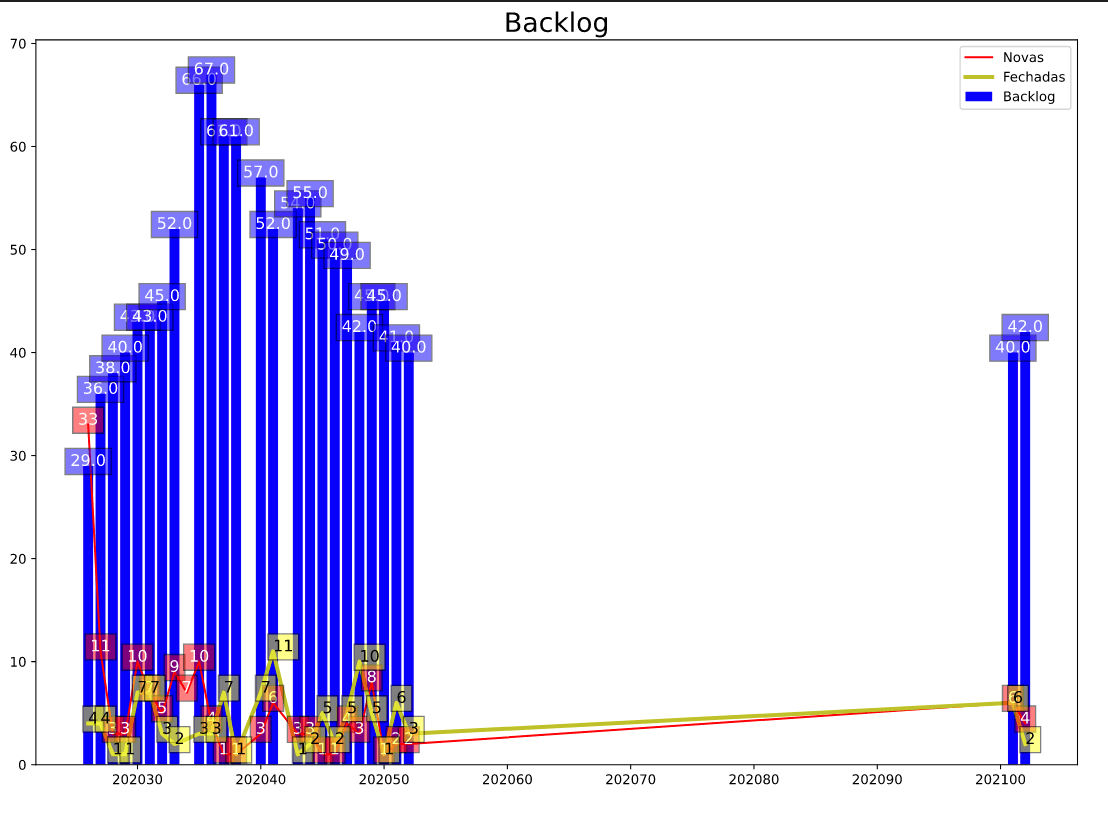# Matplotlib axes only with values on Pandas Dataframe

I'm working on a backlog chart since last year, and now with the new year,and now I'm facing this issue:I had to multiply the number of the year to keep the X axis keep rolling to the right. But after that, I got this blanked interval on X axis from 202052 (concatenate year + week of the year number) until 202099~ .

My indexes doesn't have these values. As below :

``````(Int64Index([202026, 202027, 202028, 202029, 202030, 202031, 202032, 202033,
202035, 202036, 202037, 202038, 202040, 202041, 202043, 202044,
202045, 202046, 202047, 202048, 202049, 202050, 202051, 202052,
202101, 202102],
dtype='int64'),
Int64Index([202026, 202027, 202028, 202029, 202030, 202031, 202032, 202033,
202034, 202035, 202036, 202037, 202038, 202040, 202041, 202043,
202044, 202045, 202046, 202047, 202048, 202049, 202050, 202051,
202052, 202101, 202102],
dtype='int64'),
Int64Index([202026, 202027, 202028, 202029, 202030, 202031, 202032, 202033,
202034, 202035, 202036, 202037, 202038, 202040, 202041, 202043,
202044, 202045, 202046, 202047, 202048, 202049, 202050, 202051,
202052, 202101, 202102],
dtype='int64'))

``````

How can I drop these values?

Thank you!

``````
import matplotlib.pyplot as plt
import pandas as pd
from datetime import datetime, timedelta
from matplotlib.lines import Line2D
import matplotlib.dates as mdates
import matplotlib.cbook as cbook
from matplotlib.ticker import MaxNLocator

%matplotlib inline

df.columns = df.columns.str.replace(' ', '')

df['CreatedDate'] = pd.to_datetime(df['CreatedDate'])
df['CompletedDate'] = pd.to_datetime(df['CompletedDate'])
df['DayCompleted'] = df['CompletedDate'].dt.dayofweek
df['DayCreated'] = df['CreatedDate'].dt.dayofweek
df['WeekCreated'] = df['CreatedDate'].dt.isocalendar().week
df['WeekCompleted'] = df['CompletedDate'].dt.isocalendar().week
df['YearCreated'] = df['CreatedDate'].dt.year
df['YearCompleted'] = df['CompletedDate'].dt.year
df['firstCompletedDate'] = df.CompletedDate - df.DayCompleted * timedelta(days=1)
df['firstCreatedDate'] = df.CreatedDate - df.DayCreated * timedelta(days=1)

df['YearWeekCreated'] = df['YearCreated']*100 + df['WeekCreated']
df['YearWeekCompleted'] = df['YearCompleted']*100 + df['WeekCompleted']

df_done = df[df['Progress'] == 'Completed']
df_open = df[df['Progress'] != 'Completed']
df_todo = df[df['BucketName'] == 'To do']
df_doing = df[df['BucketName'] == 'Doing']
df_consult = df[df['BucketName'] == 'Em andamento RSM']
df_open['Priority'].value_counts().sort_index()
df['Priority'].sort_index()

df_backlog_created = df['YearWeekCreated'].value_counts().sort_index()
df_backlog_completed = df['YearWeekCompleted'].value_counts().sort_index()
df_backlog = df_backlog_created.cumsum() - df_backlog_completed.cumsum()

#============================================================================

qtd_created = df['YearWeekCreated'].value_counts().sort_index()
idx_created = qtd_created.index
qtd_completed = df['YearWeekCompleted'].value_counts().sort_index()
idx_completed = qtd_completed.index
qtd_backlog = df_backlog
idx_backlog = qtd_backlog.index

idx_completed = idx_completed.astype(int)

fig, ax = plt.subplots(figsize=(14,10))

#plt.figure(figsize=(14,10))
ax.bar(idx_backlog, qtd_backlog, label="Backlog", color="b")

x=[1,2,3]
y=[9,8,7]

for a,b in zip(idx_created, qtd_created):
plt.text(a, b, str(b), fontsize=12, color='w', bbox=dict(facecolor='red', alpha=0.5), horizontalalignment='center')

for a,b in zip(idx_backlog, qtd_backlog):
plt.text(a, b, str(b), fontsize=12, color='w', bbox=dict(facecolor='blue', alpha=0.5), horizontalalignment='center')

for a,b in zip(idx_completed, qtd_completed):
plt.text(a, b, str(b), fontsize=12, color='black', bbox=dict(facecolor='yellow', alpha=0.5))

plt.title('Backlog', fontsize= 20)

``````